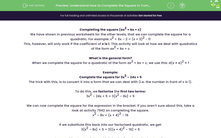# Understand How to Complete the Square in Complex Quadratics

In this worksheet, students will practise completing the square for quadratics.Key stage:  KS 4

Year:  GCSE

GCSE Subjects:   Maths

GCSE Boards:   AQA, Eduqas, OCR, Pearson Edexcel,

Curriculum topic:   Algebra

Curriculum subtopic:   Notation, Vocabulary and Manipulation Algebraic Expressions

Difficulty level:#### Worksheet Overview

Completing the square (ax+ bx + c)

We have shown in previous worksheets for the other levels, that we can complete the square for a quadratic. For example: x2 + 6x – 2 = (x + 3)2 - 11

This, however, will only work if the coefficient of x is 1. This activity will look at how we deal with quadratics of the form ax2 + bx + c

What is the general form?

When we complete the square for a quadratic of the form ax2 + bx + c, we use this: d(x ± e)2 ± f

Example:

Complete the square for 3x2 – 24x + 5

The trick with this, is to convert it into a form that we can deal with (i.e. the number in front of x is 1).

To do this, we factorise the first two terms:

3x2 – 24x + 5 = 3{x2 – 8x} + 5

We can now complete the square for the expression in the bracket. If you aren’t sure about this, take a look at activity 7942 on completing the square.

x2 – 8x = (x + 4)2 – 16

If we substitute this back into our factorised quadratic, we get:

3{x2 – 8x} + 5 = 3{(x + 4)2 – 16} + 5

Our penultimate step is to multiply by the 3 we factorised out earlier:

3{(x + 4)2 – 16} + 5 = 3(x + 4)2 –  48 + 5

And the final step is to collect the like terms:

3(x + 4)2 – 48 + 5 = 3(x + 4)2 – 43

There's lots to remember here, so have another look through the example before attempting the questions.

### What is EdPlace?

We're your National Curriculum aligned online education content provider helping each child succeed in English, maths and science from year 1 to GCSE. With an EdPlace account you’ll be able to track and measure progress, helping each child achieve their best. We build confidence and attainment by personalising each child’s learning at a level that suits them.

Get started